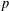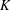Hostname: page-component-7d684dbfc8-4nnqn Total loading time: 0 Render date: 2023-09-26T14:07:37.429Z Has data issue: false Feature Flags: { "corePageComponentGetUserInfoFromSharedSession": true, "coreDisableEcommerce": false, "coreDisableSocialShare": false, "coreDisableEcommerceForArticlePurchase": false, "coreDisableEcommerceForBookPurchase": false, "coreDisableEcommerceForElementPurchase": false, "coreUseNewShare": true, "useRatesEcommerce": true } hasContentIssue false

## Abstract

Core share and HTML view are not possible as this article does not have html content. However, as you have access to this content, a full PDF is available via the ‘Save PDF’ action button.

In this paper we study the variation of the$p$-Selmer rank parities of$p$-twists of a principally polarized Abelian variety over an arbitrary number field$K$ and show, under certain assumptions, that this parity is periodic with an explicit period. Our result applies in particular to principally polarized Abelian varieties with full$K$-rational$p$-torsion subgroup, arbitrary elliptic curves, and Jacobians of hyperelliptic curves. Assuming the Shafarevich–Tate conjecture, our result allows one to classify the rank parities of all quadratic twists of an elliptic or hyperelliptic curve after a finite calculation.

## MSC classification

Type
Research Article
Information
© 2016 Australian Mathematical Publishing Association Inc.

## References

Goldfeld, D., Conjectures on Elliptic Curves over Quadratic Fields, Lecture Notes in Mathematics 751 (Springer, New York, 1979), 108118.Google Scholar
Kane, D. M., ‘On the ranks of the 2-Selmer groups of twists of a given elliptic curve’, Algebra & Number Theory 5 (2013), 12531297.CrossRefGoogle Scholar
Klagsbrun, Z., Mazur, B. and Rubin, K., ‘Disparity in Selmer ranks of quadratic twists of elliptic curves’, Ann. of Math. (2) 178(1) (2013), 287320.CrossRefGoogle Scholar
Kramer, K., ‘Arithmetic of elliptic curves upon quadratic extensions’, Trans. Amer. Math. Soc. 264 (1981), 121135.CrossRefGoogle Scholar
Mazur, B., ‘Rational points of abelian varieties with values in towers of number fields’, Invent. Math. 18(3–4) (1972), 183266.CrossRefGoogle Scholar
Mazur, B. and Rubin, K., ‘Finding large Selmer rank via an arithmetic theory of local constants’, Ann. of Math. (2) 166(2) (2007), 579612.CrossRefGoogle Scholar
Mazur, B. and Rubin, K., ‘Ranks of twists of elliptic curves and Hilbert’s tenth problem’, Invent. Math. 181(3) (2010), 541575.CrossRefGoogle Scholar
Mazur, B., Rubin, K. and Silverberg, A., ‘Twisting commutative algebraic groups’, J. Algebra 314(1) (2007), 419438.CrossRefGoogle Scholar
Milne, J. S., Arithmetic Duality Theorems, Perspectives in Mathematics 1 (Academic Press, Boston, 1986).Google Scholar
Poonen, B. and Rains, E., ‘Random maximal isotropic subspaces and Selmer groups’, J. Amer. Math. Soc. 25(1) (2012), 245269.CrossRefGoogle Scholar
Poonen, B. and Stoll, M., ‘The Cassels–Tate pairing on polarized Abelian varieties’, Ann. of Math. (2) 150(3) (1999), 11091149.CrossRefGoogle Scholar
Rubin, K., Euler Systems, Annals of Mathematics Studies (Princeton University Press, Princeton, NJ, 2000).CrossRefGoogle Scholar
Stein, W. A. et al. , Sage Mathematics Software (Version 6.4.1). The Sage Developement Team (2014), http://www.sagemath.org.Google Scholar
Swinnerton-Dyer, P., ‘The effect of twisting on the 2-Selmer group’, Math. Proc. Cambridge Philos. Soc. 145(3) (2008), 513526.CrossRefGoogle Scholar
The PARI Group, Bordeaux (Version 2.7.0) (2014), http://pari.math.u-bordeaux.fr/.Google Scholar
Yu, M., ‘Selmer ranks of twists of hyperelliptic curves and superelliptic curves’. PhD Thesis, UC Irvine.Google Scholar